# RD Sharma Solutions for Class 10 Maths Chapter 7 Statistics Exercise 7.5

In statistics, the mode is one important parameter. This exercise completely deals with finding mode/ modal value of the given continuous frequency distribution. Suitable methods are introduced for computing the modal value, and this can be clearly understood in the RD Sharma Solutions Class 10. If you want to get the grip over finding mode, the RD Sharma Solutions for Class 10 Maths Chapter 7 Statistics Exercise 7.5 PDF is available for free to download.

## RD Sharma Solutions for Class 10 Maths Chapter 7 Statistics Exercise 7.5 Download PDF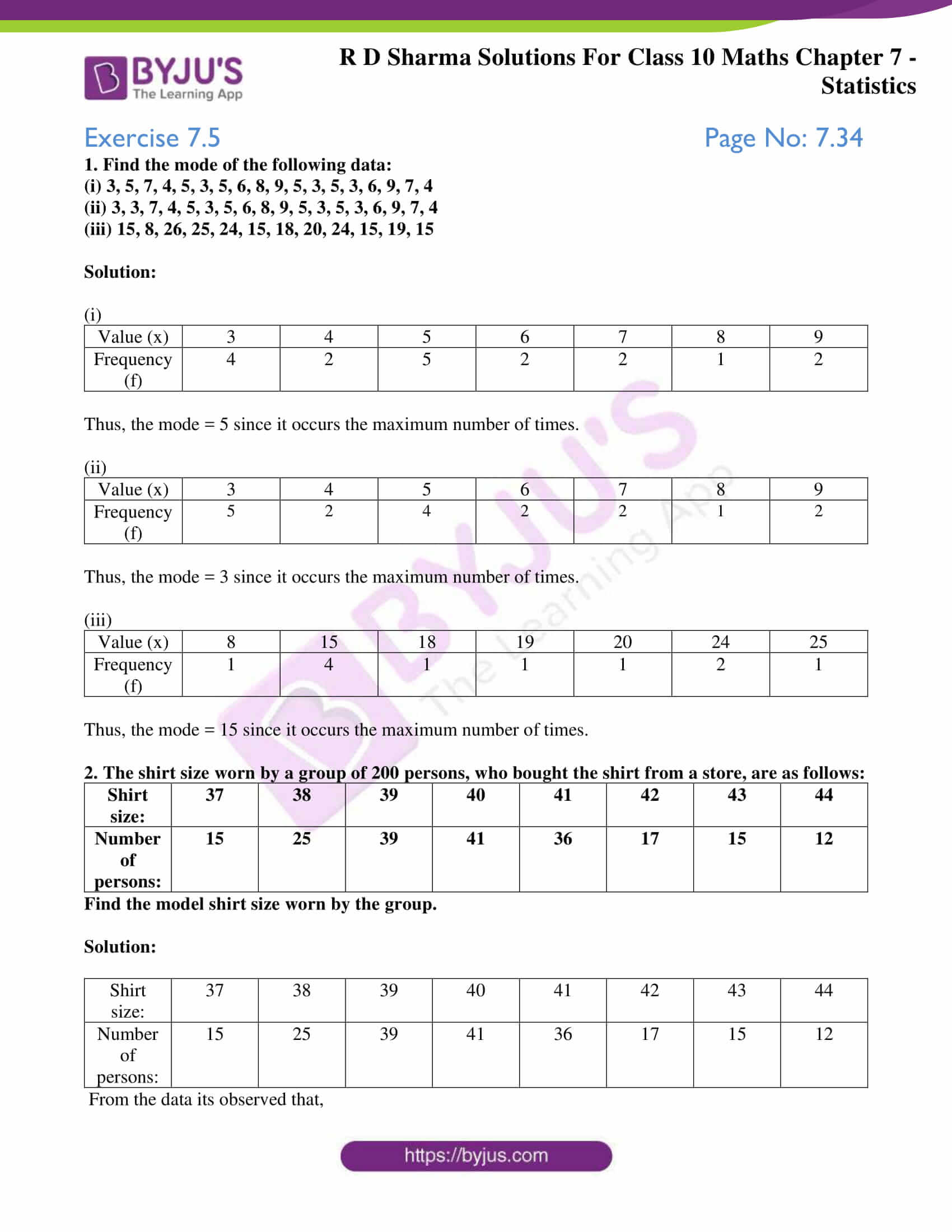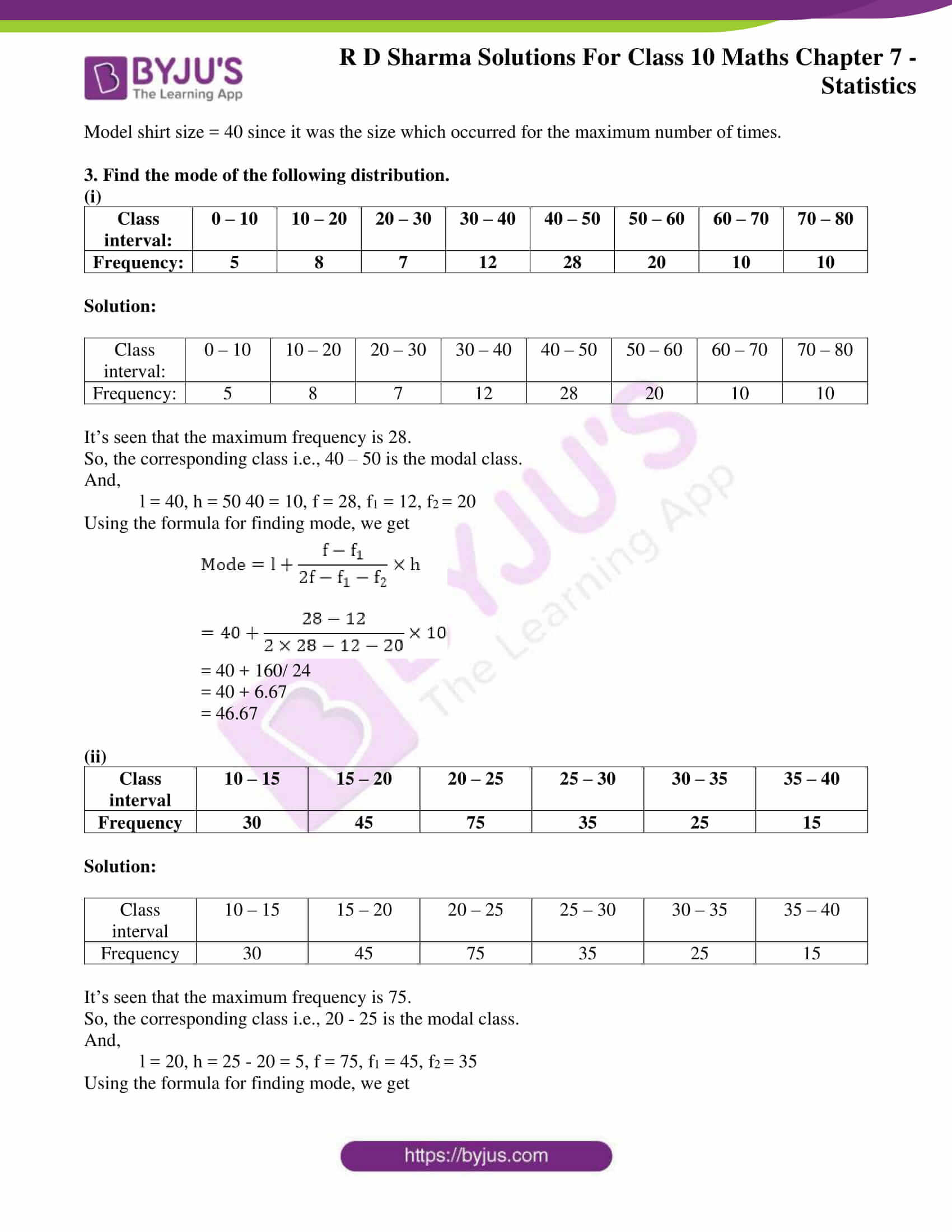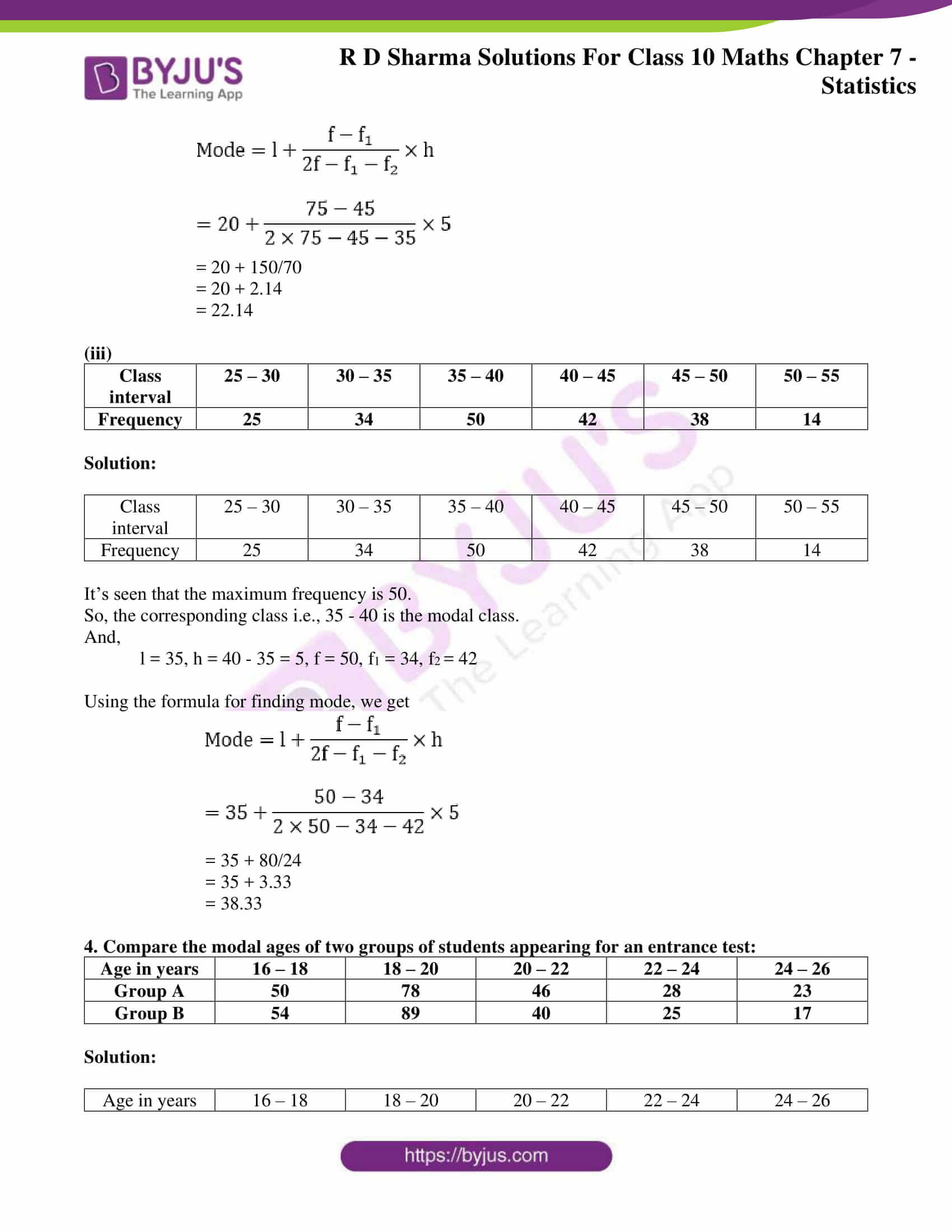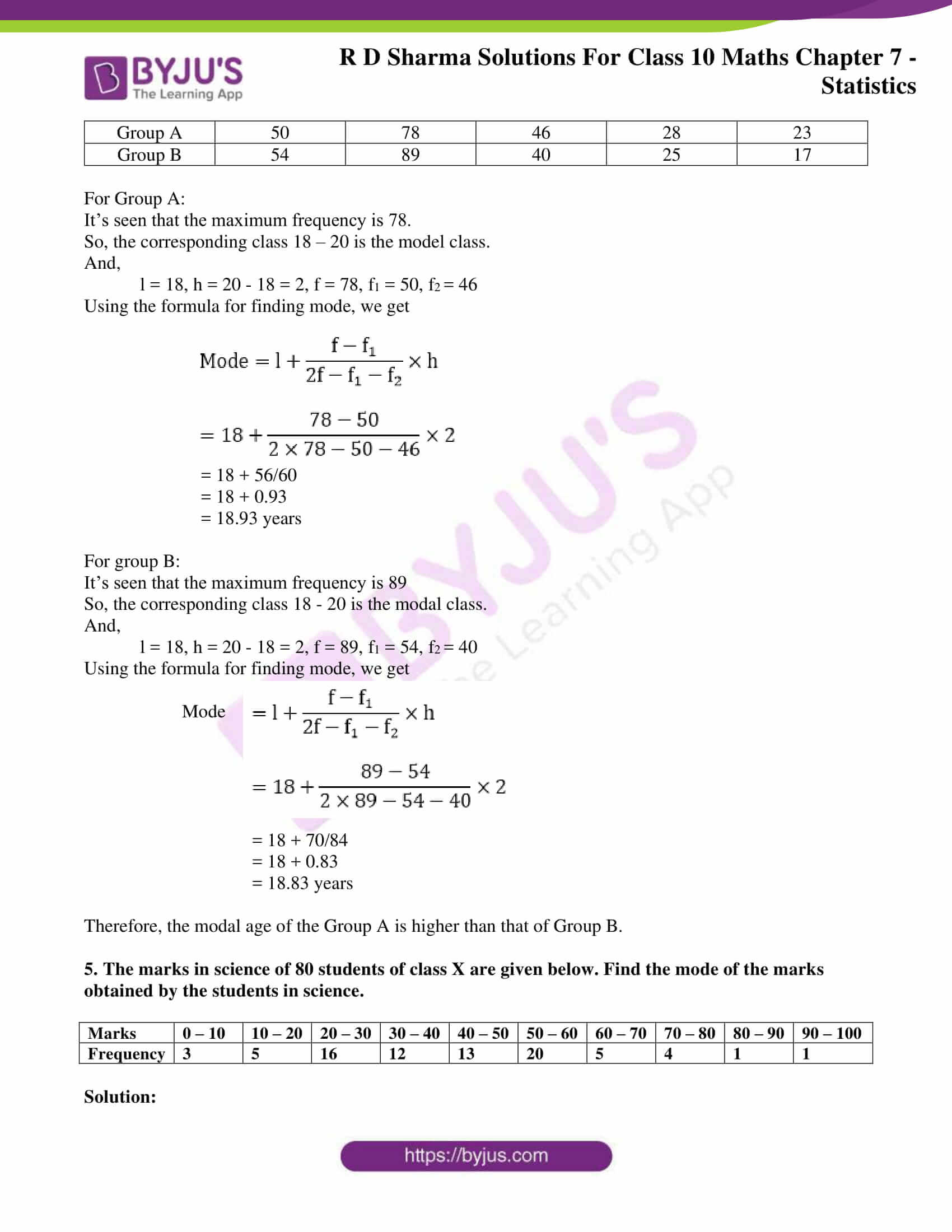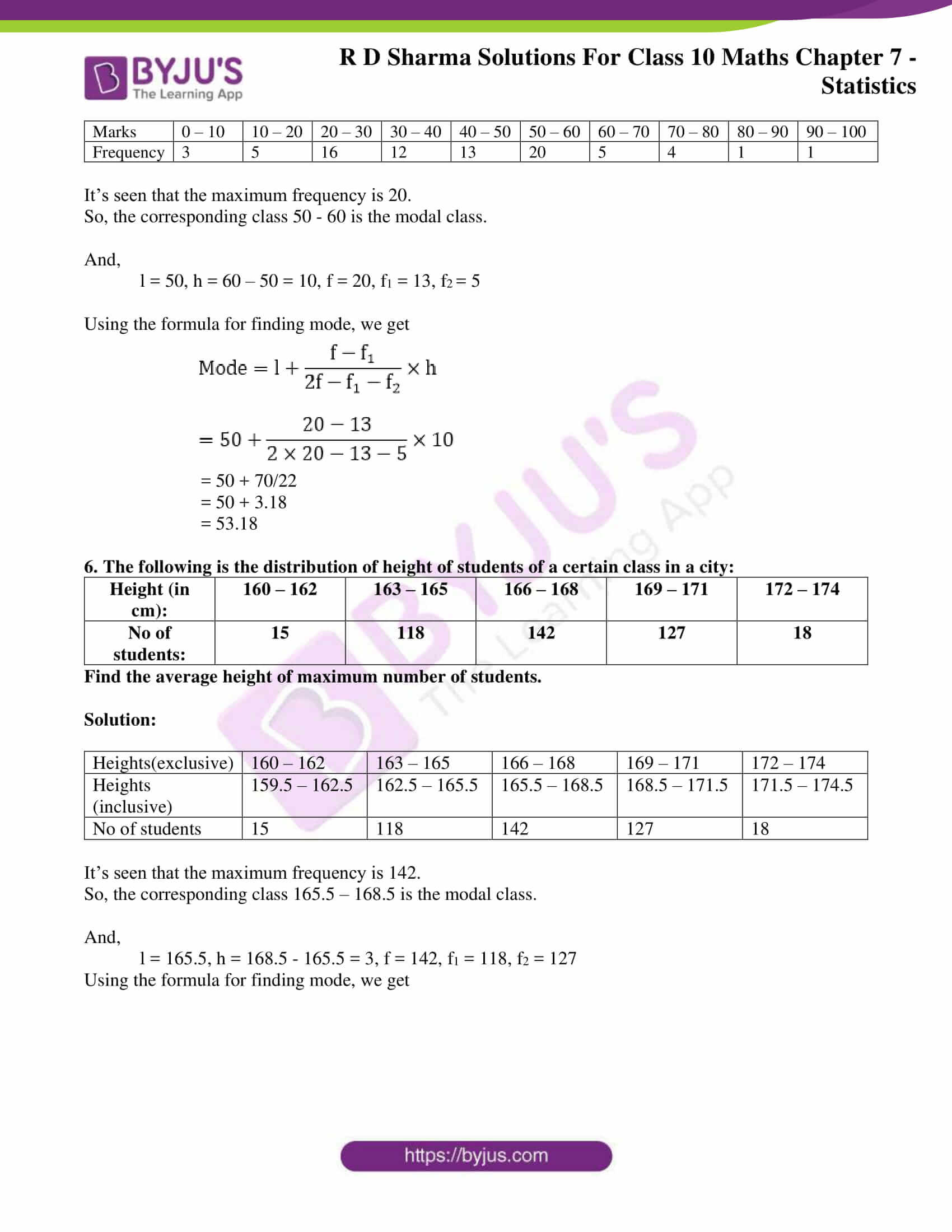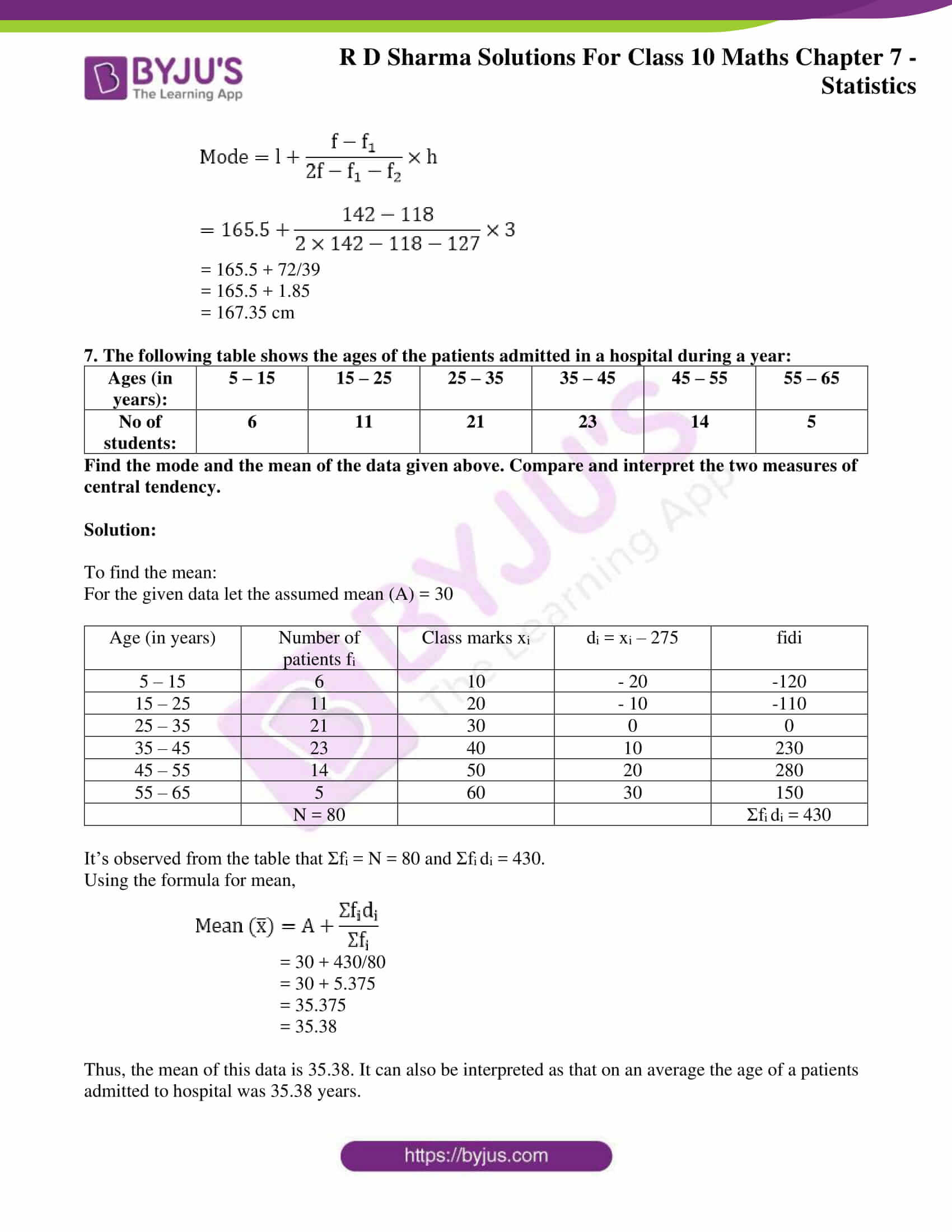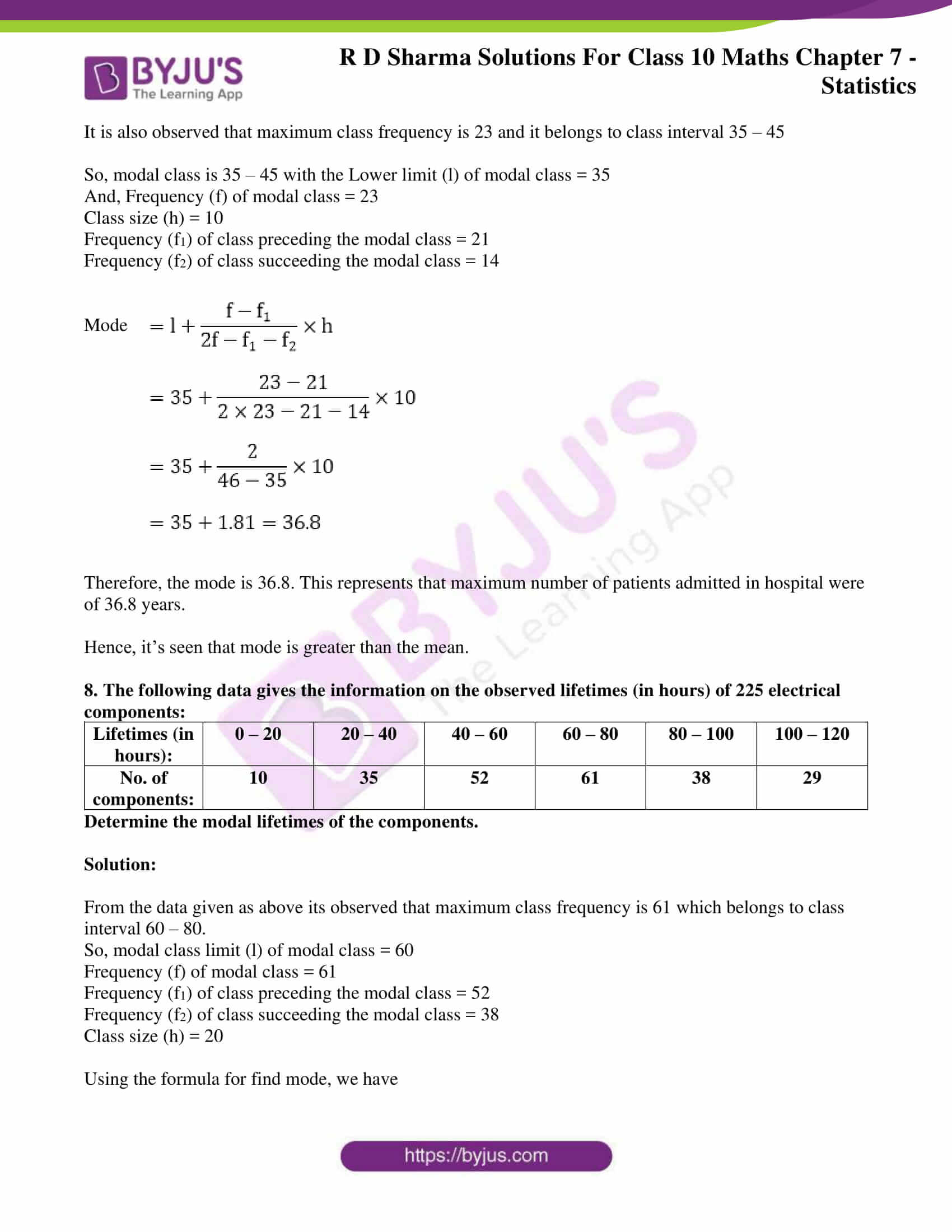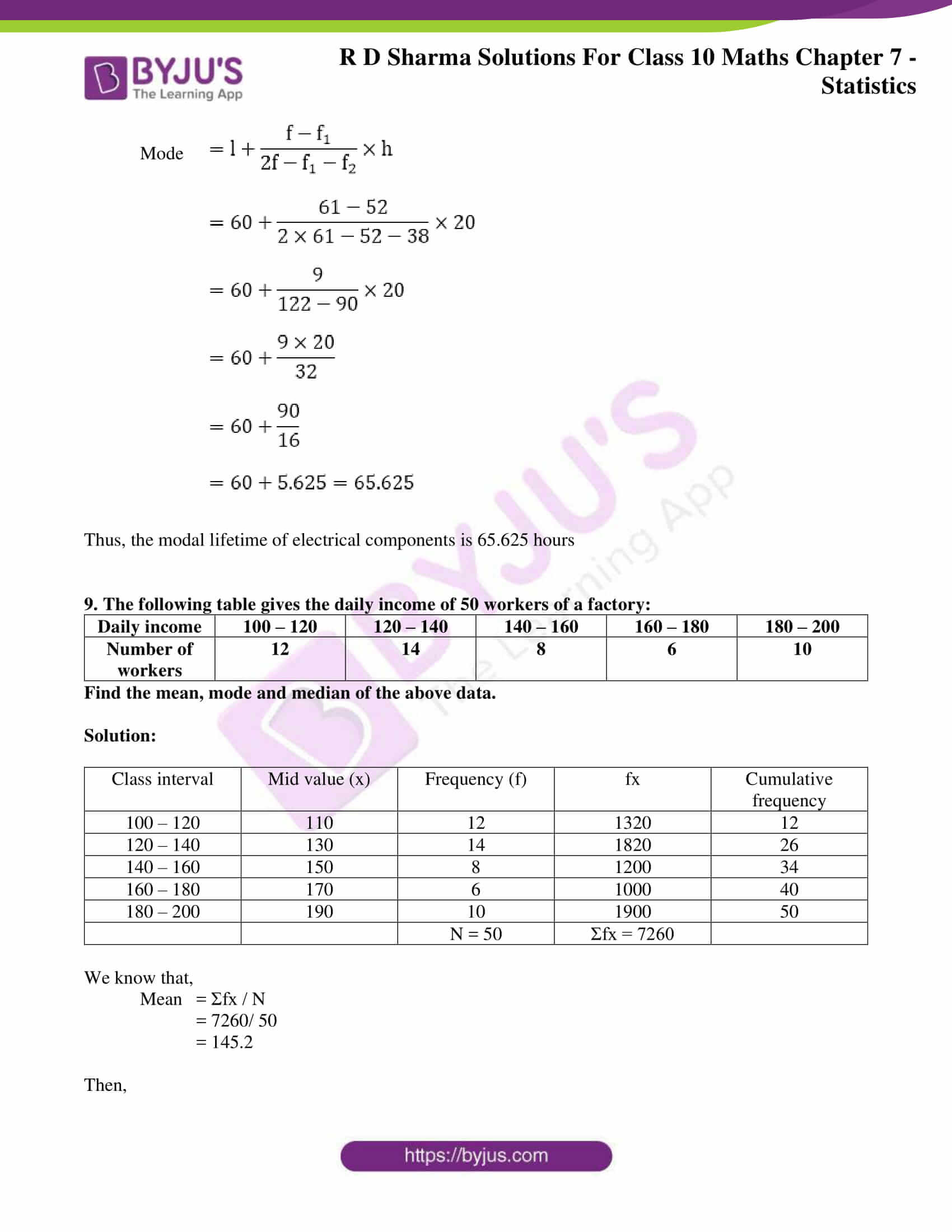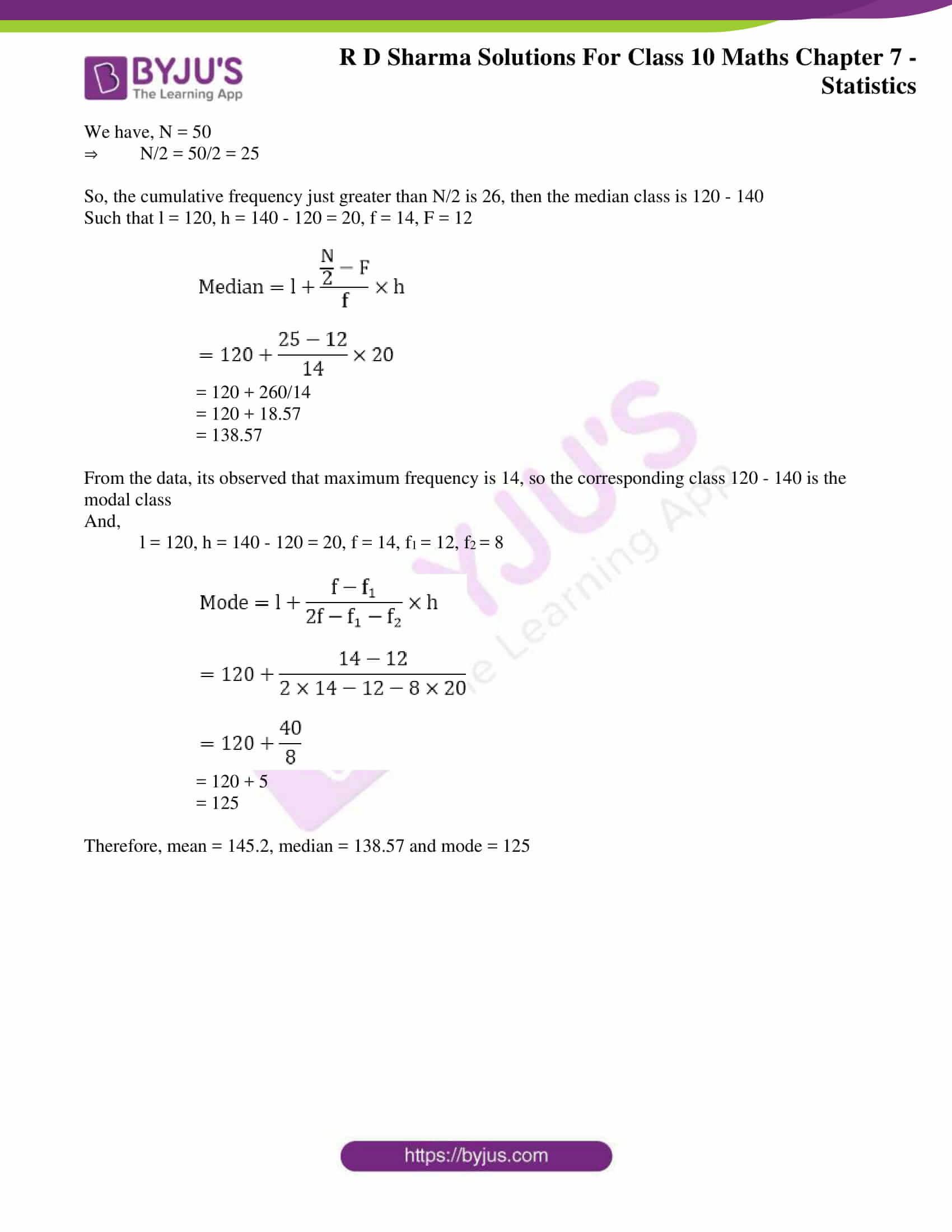### Access RD Sharma Solutions for Class 10 Maths Chapter 7 Statistics Exercise 7.5

1. Find the mode of the following data:

(i) 3, 5, 7, 4, 5, 3, 5, 6, 8, 9, 5, 3, 5, 3, 6, 9, 7, 4

(ii) 3, 3, 7, 4, 5, 3, 5, 6, 8, 9, 5, 3, 5, 3, 6, 9, 7, 4

(iii) 15, 8, 26, 25, 24, 15, 18, 20, 24, 15, 19, 15

Solution:

(i)

 Value (x) 3 4 5 6 7 8 9 Frequency (f) 4 2 5 2 2 1 2

Thus, the mode = 5 since it occurs the maximum number of times.

(ii)

 Value (x) 3 4 5 6 7 8 9 Frequency (f) 5 2 4 2 2 1 2

Thus, the mode = 3 since it occurs the maximum number of times.

(iii)

 Value (x) 8 15 18 19 20 24 25 Frequency (f) 1 4 1 1 1 2 1

Thus, the mode = 15 since it occurs the maximum number of times.

2. The shirt size worn by a group of 200 persons, who bought the shirt from a store, are as follows:

 Shirt size: 37 38 39 40 41 42 43 44 Number of persons: 15 25 39 41 36 17 15 12

Find the model shirt size worn by the group.

Solution:

 Shirt size: 37 38 39 40 41 42 43 44 Number of persons: 15 25 39 41 36 17 15 12

From the data its observed that,

Model shirt size = 40 since it was the size which occurred for the maximum number of times.

3. Find the mode of the following distribution.

(i)

 Class interval: 0 – 10 10 – 20 20 – 30 30 – 40 40 – 50 50 – 60 60 – 70 70 – 80 Frequency: 5 8 7 12 28 20 10 10

Solution:

 Class interval: 0 – 10 10 – 20 20 – 30 30 – 40 40 – 50 50 – 60 60 – 70 70 – 80 Frequency: 5 8 7 12 28 20 10 10

It’s seen that the maximum frequency is 28.

So, the corresponding class i.e., 40 – 50 is the modal class.

And,

l = 40, h = 50 40 = 10, f = 28, f1 = 12, f= 20

Using the formula for finding mode, we get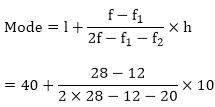= 40 + 160/ 24

= 40 + 6.67

= 46.67

(ii)

 Class interval 10 – 15 15 – 20 20 – 25 25 – 30 30 – 35 35 – 40 Frequency 30 45 75 35 25 15

Solution:

 Class interval 10 – 15 15 – 20 20 – 25 25 – 30 30 – 35 35 – 40 Frequency 30 45 75 35 25 15

It’s seen that the maximum frequency is 75.

So, the corresponding class i.e., 20 – 25 is the modal class.

And,

l = 20, h = 25 – 20 = 5, f = 75, f1 = 45, f= 35

Using the formula for finding mode, we get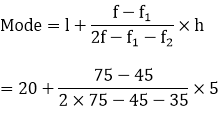= 20 + 150/70

= 20 + 2.14

= 22.14

(iii)

 Class interval 25 – 30 30 – 35 35 – 40 40 – 45 45 – 50 50 – 55 Frequency 25 34 50 42 38 14

Solution:

 Class interval 25 – 30 30 – 35 35 – 40 40 – 45 45 – 50 50 – 55 Frequency 25 34 50 42 38 14

It’s seen that the maximum frequency is 50.

So, the corresponding class i.e., 35 – 40 is the modal class.

And,

l = 35, h = 40 – 35 = 5, f = 50, f1 = 34, f= 42

Using the formula for finding mode, we get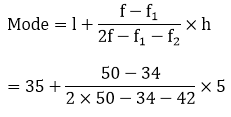= 35 + 80/24

= 35 + 3.33

= 38.33

4. Compare the modal ages of two groups of students appearing for an entrance test:

 Age in years 16 – 18 18 – 20 20 – 22 22 – 24 24 – 26 Group A 50 78 46 28 23 Group B 54 89 40 25 17

Solution:

 Age in years 16 – 18 18 – 20 20 – 22 22 – 24 24 – 26 Group A 50 78 46 28 23 Group B 54 89 40 25 17

For Group A:

It’s seen that the maximum frequency is 78.

So, the corresponding class 18 – 20 is the model class.

And,

l = 18, h = 20 – 18 = 2, f = 78, f1 = 50, f= 46

Using the formula for finding mode, we get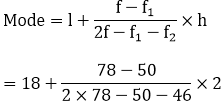= 18 + 56/60

= 18 + 0.93

= 18.93 years

For group B:

It’s seen that the maximum frequency is 89

So, the corresponding class 18 – 20 is the modal class.

And,

l = 18, h = 20 – 18 = 2, f = 89, f1 = 54, f= 40

Using the formula for finding mode, we get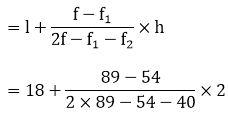Mode

= 18 + 70/84

= 18 + 0.83

= 18.83 years

Therefore, the modal age of the Group A is higher than that of Group B.

5. The marks in science of 80 students of class X are given below. Find the mode of the marks obtained by the students in science.

 Marks 0 – 10 10 – 20 20 – 30 30 – 40 40 – 50 50 – 60 60 – 70 70 – 80 80 – 90 90 – 100 Frequency 3 5 16 12 13 20 5 4 1 1

Solution:

 Marks 0 – 10 10 – 20 20 – 30 30 – 40 40 – 50 50 – 60 60 – 70 70 – 80 80 – 90 90 – 100 Frequency 3 5 16 12 13 20 5 4 1 1

It’s seen that the maximum frequency is 20.

So, the corresponding class 50 – 60 is the modal class.

And,

l = 50, h = 60 – 50 = 10, f = 20, f1 = 13, f= 5

Using the formula for finding mode, we get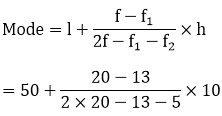= 50 + 70/22

= 50 + 3.18

= 53.18

6. The following is the distribution of height of students of a certain class in a city:

 Height (in cm): 160 – 162 163 – 165 166 – 168 169 – 171 172 – 174 No of students: 15 118 142 127 18

Find the average height of maximum number of students.

Solution:

 Heights(exclusive) 160 – 162 163 – 165 166 – 168 169 – 171 172 – 174 Heights (inclusive) 159.5 – 162.5 162.5 – 165.5 165.5 – 168.5 168.5 – 171.5 171.5 – 174.5 No of students 15 118 142 127 18

It’s seen that the maximum frequency is 142.

So, the corresponding class 165.5 – 168.5 is the modal class.

And,

l = 165.5, h = 168.5 – 165.5 = 3, f = 142, f1 = 118, f2 = 127

Using the formula for finding mode, we get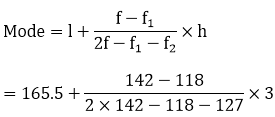= 165.5 + 72/39

= 165.5 + 1.85

= 167.35 cm

7. The following table shows the ages of the patients admitted in a hospital during a year:

 Ages (in years): 5 – 15 15 – 25 25 – 35 35 – 45 45 – 55 55 – 65 No of students: 6 11 21 23 14 5

Find the mode and the mean of the data given above. Compare and interpret the two measures of central tendency.

Solution:

To find the mean:

For the given data let the assumed mean (A) = 30

 Age (in years) Number of patients fi Class marks xi di = xi – 275 fidi 5 – 15 6 10 – 20 -120 15 – 25 11 20 – 10 -110 25 – 35 21 30 0 0 35 – 45 23 40 10 230 45 – 55 14 50 20 280 55 – 65 5 60 30 150 N = 80 Σfi di = 430

It’s observed from the table that Σfi = N = 80 and Σfdi = 430.

Using the formula for mean,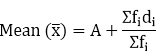= 30 + 430/80

= 30 + 5.375

= 35.375

= 35.38

Thus, the mean of this data is 35.38. It can also be interpreted as that on an average the age of a patients admitted to hospital was 35.38 years.

It is also observed that maximum class frequency is 23 and it belongs to class interval 35 – 45

So, modal class is 35 – 45 with the Lower limit (l) of modal class = 35

And, Frequency (f) of modal class = 23

Class size (h) = 10

Frequency (f1) of class preceding the modal class = 21

Frequency (f2) of class succeeding the modal class = 14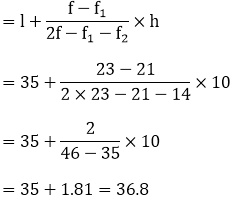Mode

Therefore, the mode is 36.8. This represents that maximum number of patients admitted in hospital were of 36.8 years.

Hence, it’s seen that mode is greater than the mean.

8. The following data gives the information on the observed lifetimes (in hours) of 225 electrical components:

 Lifetimes (in hours): 0 – 20 20 – 40 40 – 60 60 – 80 80 – 100 100 – 120 No. of components: 10 35 52 61 38 29

Determine the modal lifetimes of the components.

Solution:

From the data given as above its observed that maximum class frequency is 61 which belongs to class interval 60 – 80.

So, modal class limit (l) of modal class = 60

Frequency (f) of modal class = 61

Frequency (f1) of class preceding the modal class = 52

Frequency (f2) of class succeeding the modal class = 38

Class size (h) = 20

Using the formula for find mode, we have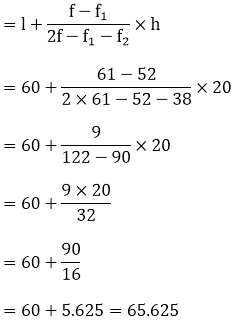Mode

Thus, the modal lifetime of electrical components is 65.625 hours

9. The following table gives the daily income of 50 workers of a factory:

 Daily income 100 – 120 120 – 140 140 – 160 160 – 180 180 – 200 Number of workers 12 14 8 6 10

Find the mean, mode and median of the above data.

Solution:

 Class interval Mid value (x) Frequency (f) fx Cumulative frequency 100 – 120 110 12 1320 12 120 – 140 130 14 1820 26 140 – 160 150 8 1200 34 160 – 180 170 6 1000 40 180 – 200 190 10 1900 50 N = 50 Σfx = 7260

We know that,

Mean = Σfx / N

= 7260/ 50

= 145.2

Then,

We have, N = 50

⇒ N/2 = 50/2 = 25

So, the cumulative frequency just greater than N/2 is 26, then the median class is 120 – 140

Such that l = 120, h = 140 – 120 = 20, f = 14, F = 12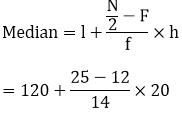= 120 + 260/14

= 120 + 18.57

= 138.57

From the data, its observed that maximum frequency is 14, so the corresponding class 120 – 140 is the modal class

And,

l = 120, h = 140 – 120 = 20, f = 14, f= 12, f= 8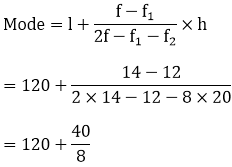= 120 + 5

= 125

Therefore, mean = 145.2, median = 138.57 and mode = 125